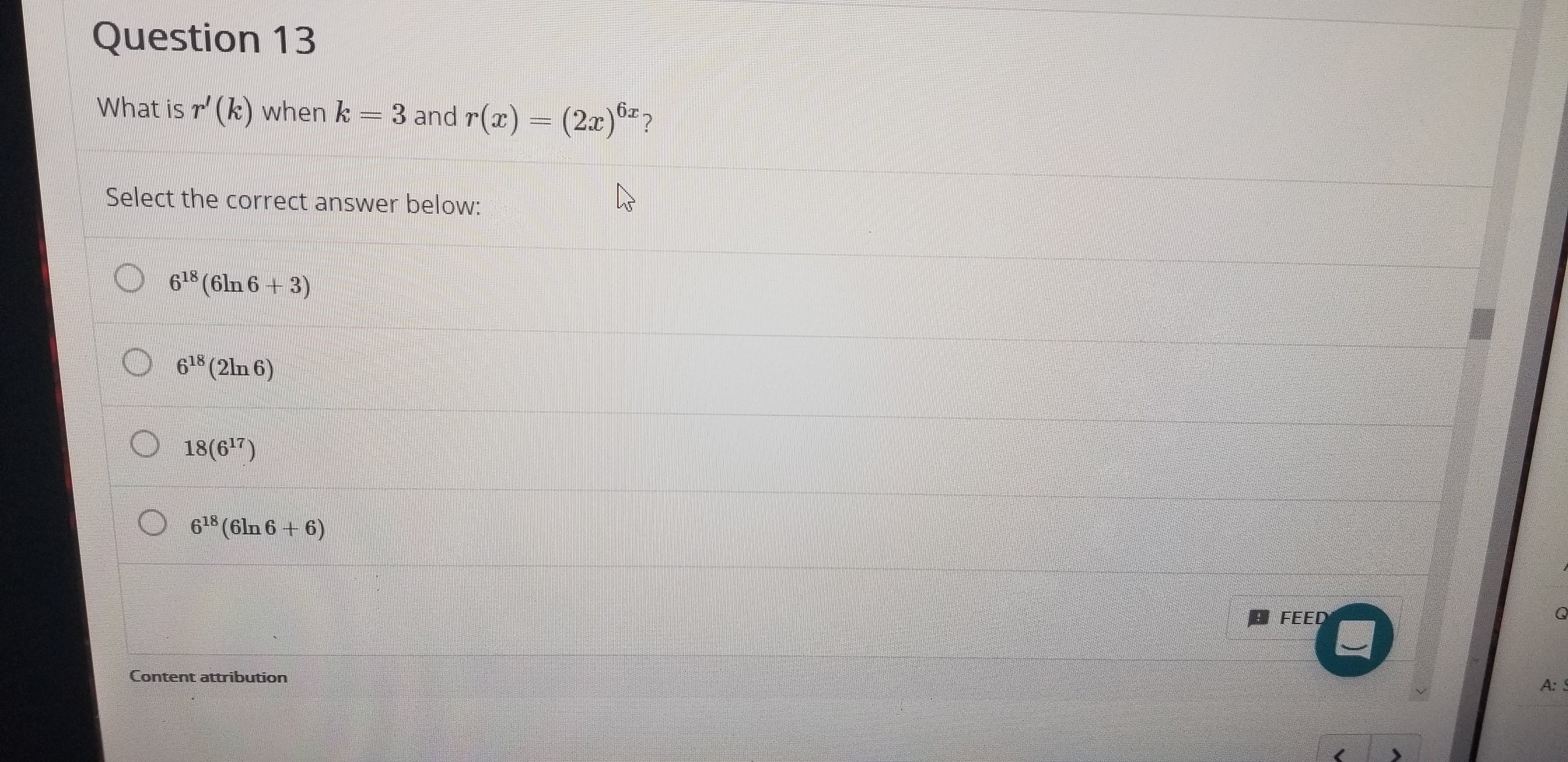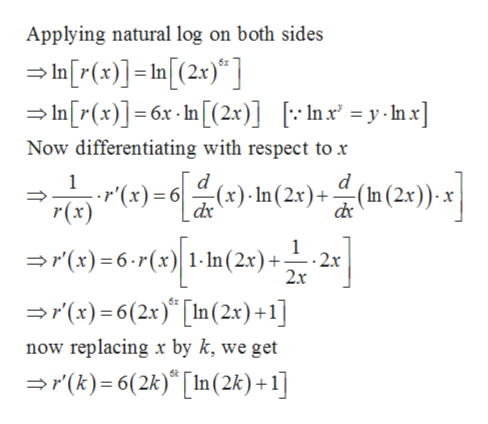# Question 13What is r' (k) when k3 and r(x)(2)6Select the correct answer below:618 (6ln 6+ 3)O 618 (2ln 6)O 18(67)O 618 (6ln 6 + 6)FEEDA: SContent attribution

Question
45 viewshelp_outlineImage TranscriptioncloseQuestion 13 What is r' (k) when k 3 and r(x) (2)6 Select the correct answer below: 618 (6ln 6+ 3) O 618 (2ln 6) O 18(67) O 618 (6ln 6 + 6) FEED A: S Content attribution fullscreen
check_circle

Step 1

Given,

Step 2

Differentiating by using logarithmic diff...help_outlineImage TranscriptioncloseApplying natural log on both sides In[r(x)]=In[(2x)"] In[r(x)]=6x-n[(2x)] [Inx = y-mx] Now differentiating with respect to x 1 r'(x) 6(x). In ( 2.x) + - (n (2x) x 1 2x 2x r(x)=6-P(x) 1-In(2x)+ (x)6(2x) [n (2x) +1] now replacing x by k, we get r(k)6(2k) [In (2k) + 1] fullscreen

### Want to see the full answer?

See Solution

#### Want to see this answer and more?

Solutions are written by subject experts who are available 24/7. Questions are typically answered within 1 hour.*

See Solution
*Response times may vary by subject and question.
Tagged in

### Derivative